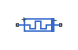# Memristor

Ideal memristor with nonlinear dopant drift approach

• Library:
• Simscape / Foundation Library / Electrical / Electrical Elements

•## Description

This block allows you to model an ideal memristor with a nonlinear dopant drift approach. The behavior of memristor is similar to a resistor, except that its resistance (also called memristance) is a function of the current that has passed through the device. The memristance is defined by two states, A and B, with some fraction of the device in one of those states at a given time.

The nonlinear dopant drift model, , is described with the following equations:

V = M·I

M = ξ·RA+(1 – ξ)·RB

`$\frac{d\xi }{dt}=\frac{I}{{Q}_{0}}{F}_{p}\left(\xi \right)$`

where

• V is the voltage across the memristor.

• M is the memristance.

• I is the current entering the + terminal.

• RA and RB are the resistances of the A and B states, respectively.

• ξ is the fraction of the memristor in state A. A positive current from the + terminal to the - terminal increases ξ. Similarly, a positive current from the - terminal to the + terminal decreases ξ. The value of ξ is bounded by 0 and 1.

• t is time.

• Q0 is the total charge required to make the memristor transition from being fully in one state to being fully in the other state.

• Fp(ξ) is a "window" function, which keeps ξ in the window between 1 and 0, and therefore gives zero drift at the boundaries of the device.

The window function is

Fp(ξ) = 1 – (2ξ – 1)2p

where p is a positive integer. This function is modified when ξ is close to either 0 or 1, to improve numerical stability.

### Variables

To set the priority and initial target values for the block variables prior to simulation, use the Initial Targets section in the block dialog box or Property Inspector. For more information, see Set Priority and Initial Target for Block Variables.

Nominal values provide a way to specify the expected magnitude of a variable in a model. Using system scaling based on nominal values increases the simulation robustness. Nominal values can come from different sources, one of which is the Nominal Values section in the block dialog box or Property Inspector. For more information, see Modify Nominal Values for a Block Variable.

## Ports

### Conserving

expand all

Electrical conserving port associated with the memristor positive terminal.

Electrical conserving port associated with the memristor negative terminal.

## Parameters

expand all

The resistance if the entire memristor is in state A, that is, if ξ = 1. The value should be greater than 0.

The resistance if the entire memristor is in state B, that is, if ξ = 0. The value should be greater than 0.

The total charge flow that is required to transition the memristor from being fully in one state to being fully in the other state.

The initial condition for ξ at the start of the simulation. This parameter sets a high priority variable target within the block. The value should be greater than or equal to 0 and less than or equal to 1.

The exponent, p, of the window function, which keeps the value of ξ between 0 and 1.

 Joglekar, Y. N., and S. J. Wolf. "The elusive memristor: properties of basic electrical circuits." European Journal of Physics. 30, 2009, pp. 661–675.